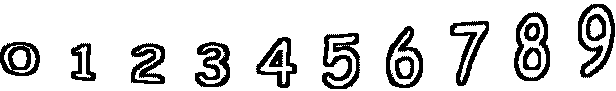3 - 1 =        3 - 0 =5 - 5 =        9 - 0 =        1 - 1 =        3 - 0 = 6 - 6 =        0 - 0 =        3 - 2 =        0 - 0 =